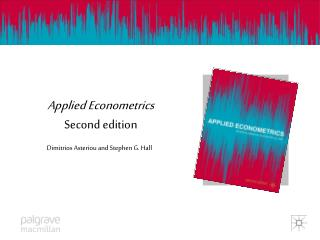# Applied Econometrics Second edition - PowerPoint PPT PresentationDownload PresentationApplied Econometrics Second edition

Applied Econometrics Second editionDownload Presentation## Applied Econometrics Second edition

- - - - - - - - - - - - - - - - - - - - - - - - - - - E N D - - - - - - - - - - - - - - - - - - - - - - - - - - -
##### Presentation Transcript

1. Applied EconometricsSecond edition Dimitrios Asteriou and Stephen G. Hall

2. DUMMY VARIABLES 1. The Nature of Qualitative Information 2. The Use of Dummy Variables 3. Special Cases of Dummy Variables 4. Dummy Variables with Multiple Categories 5. Tests for Structural Stability

3. Learning Objectives 1. Understand the various forms of possible misspecification in the CLRM. 2. Appreciate the importance and learn the consequences of omitting influential variables in the CLRM. 3. Distinguish among the wide range of functional forms and understand the meaning and interpretation of their coefficients. 4. Understand the importance of measurement errors in the data. 5. Perform misspecification tests using econometric software. 6. Understand the meaning of nested and non-nested models. 7. Be familiar with the concept of data mining and choose an appropriate econometric model.

4. The Nature of Qualitative Information Sometimes we can not obtain a set of numerical values for all the variables we want to use in a model. This is because some variables can not be quantified easily. Examples: • Gender may play a role in determining salary levels • Different ethnic groups may follow different consumption patterns • Educational levels can affect earnings from employment

5. The Nature of Qualitative Information It is easier to have dummies for cross-sectional variables, but sometimes we do have for time series as well. Examples • Changes in a political regime may affect production • A war can have an impact on economic activities • Certain days in a week or certain months in a year can have different effects in the fluctuation of stock prices • Seasonal effects are often observed in demand of various products

6. The Use of Dummy Variables Consider the following cross-sectional model: Yi=β1+β2X2i+ ui The constant term in this equation measures the mean value of Yi when X2i is equal to zero. This model assumes that the constant will be the same for all the observations in our data set. But what if we have two different subgroups (male, female for example)?

7. The Use of Dummy Variables The question here is how to quantify the information that comes from the difference in the two groups. One solution is to create a dummy variable as follows: 1 for male D = 0 for female Note that the choice of which of the two different outcomes is to be assigned the value of 1 does not alter the results.

8. The Use of Dummy Variables Entering this dummy in the equation we have the following model: Yi=β1+β2X2i+β3Di+ui Now we have two cases Di=0 Yi=β1+β2X2i+ β3(0)+ui Yi=β1+β2X2i+ui Di=1 Yi=β1+β2X2i+β3(1)+ui Yi=(β1+β3)+β2X2i+ui

9. Intercept Dummy Variable Red Points: Male Grey Points: Female

10. Intercept Dummy Variable β1+β3 β1

11. Slope Dummy Variable Consider the same case but now with the dummy affecting the slope Yi=β1+β2X2i+β3DiX2i+ui Now we have two cases Di=0 Yi=β1+β2X2i+β3(0)iX2i+ui Yi=β1+β2X2i+ui Di=1 Yi=β1+β2X2i+β3(1)iX2i+ui Yi=β1+(β2+β3)X2i+ui

12. Slope Dummy Variable

13. The Combined Effect Y Males l Females d b1 +d b1

14. Dummies with Multiple Categories Consider the case of education: D1= 1 primary; 0 otherwise D2= 1 if secondary; 0 otherwise D3= 1 if tertiary; 0 otherwise D4 = 1 if BSc; 0 otherwise D5 = 1 if MSc; 0 otherwise

15. The Plug in Solution So we estimate: Y=β1+β2X2+ a1D2+a2D3+a3D4+a4D5+u Note that one dummy (in this case D1) is excluded from the model in order to avoid dummy variable trap. Consider various cases, i.e. D2=1, D3=D4=D5=0 etc.

16. Multiple Categories

17. Using More than One Dummy GENDER (male; female) EDUC (primary, secondary, tertiary; BSc; MSc) AGE (less than 30, 30 to 40; more than 40) OCUP (unskilled, skilled, clerical, self-employed) etc The interpretation (although it seems more complicated) is the same as before

18. Seasonal Dummy Variables Depends on the frequency of the data Quarterly – 4 dummies – DQ1, DQ2, DQ3, DQ4 Monthly – 12 dummies – one for each month Daily – 5 dummies – Dmon, Dtue, Dwed etc. Again we either exclude one and include a constant (always better) or if we use all we never include a constant (dummy variable trap).

19. The Chow Test for Structural Stability Step 1: Estimate the basic regression equation. Yi=β1+β2X2i+ui for three different data sets • whole sample, n; • Period before the shock, n1; • Period after the shock, n2. Step 2: Obtain the SSR for each of the three subsets and label them SSRN, SSR1 and SSR2 respectively.

20. The Chow Test for Structural Stability Step 3: Calculate the F-statistic F Step 4: If F-statistical bigger than F-critical F(k,n-2k) then reject the null that the parameters are stable for the whole data set.

21. The Dummy Variable Test for Structural Stability The Dummy Variable Test is much better because: • A single equation is used to provide a set of the estimated coefficients for tow or more structures • Only one degree of freedom is lost for every dummy used. • A larger sample is being used for the estimation • It provides us with information regarding the exact nature of the parameter instability.